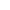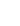Intended for healthcare professionals
Home/9. Exact probability test

# 9. Exact probability test

Sometimes in a comparison of the frequency of observations in a fourfold table the numbers are too small for the χ² test (Chapter 8). The exact probability test devised by Fisher, Irwin, and Yates (1) provides a way out of the difficulty. Tables based on it have been published – for example by Geigy (2) – showing levels at which the null hypothesis can be rejected. The method will be described here because, with the aid of a calculator, the exact probability is easily computed.
Consider the following circumstances. Some soldiers are being trained as parachutists. One rather windy afternoon 55 practice jumps take place at two localities, dropping zone A and dropping zone B. Of 15 men who jump at dropping zone A, five suffer sprained ankles, and of 40 who jump at dropping zone B, two suffer this injury. The casualty rate at dropping zone A seems unduly high, so the medical officer in charge decides to investigate the disparity. Is it a difference that might be expected by chance? If not it deserves deeper study. The figures are set out in table 9.1 . The null hypothesis is that there is no difference in the probability of injury generating the proportion of injured men at each dropping zone.The method to be described tests the exact probability of observing the particular set of frequencies in the table if the marginal totals (that is, the totals in the last row and column) are kept at their present values. But to the probability of getting this particular set of frequencies we have to add the probability of getting a set of frequencies showing greater disparity between the two dropping zones. This is because we are concerned to know the probability not only of the observed figures but also of the more extreme cases. This may seem obscure, but it ties in with the idea of calculating tail areas in the continuous case.
For convenience of computation the table is changed round to get the smallest number in the top left hand cell. We therefore begin by constructing table 9.2 from table 9.1 by transposing the upper and lower rows.The exact probability for any table is now determined from the following formula:The exclamation mark denotes “factorial” and means successive multiplication by cardinal numbers in descending series; for example 4! means 4 x 3 x 2 x 1. By convention 0! = 1. Factorial functions are available on most calculators, but care is needed not to exceed the maximum number available on the calculator. Generally factorials can be cancelled out for easy computation on a calculator (see below).
With this formula we have to find the probability attached to the observations in table 9.1 , which is equivalent to table 9.2 , and is denoted by set 2 in table 9.3 . We also have to find the probabilities attached to the more extreme cases. If ad-bc is negative, then the extreme cases are obtained by progressively decreasing cells a and d and increasing b and c by the same amount. If ad – bc is positive, then progressively increase cells a and d and decrease b and c by the same amount.(3) For table 9.2 ad – bc is negative and so the more extreme cases are sets 0 and 1.
The best way of doing this is to start with set 0. Call the probability attached to this set. Then, applying the formula, we get:This cancels down toFor computation on a calculator the factorials can be cancelled out further by removing 8! from 15! and 48! from 55! to giveWe now start from the left and divide and multiply alternately. However, on an eight digit calculator we would thereby obtain the result 0.0000317 which does not give enough significant figures. Consequently we first multiply the 15 by 1000. Alternate dividing and multiplying then gives 0.0317107. We continue to work with this figure, which isx 1000, and we now enter it in the memory while also retaining it on the display.
Remembering that we are now working with units 1000 times larger than the real units, to calculate the probability for set 1 we take the value of, multiply it by b and c from set 0, and divide it by a and d from set 1. That isThe figure foris retained on the display.
Likewise, to calculate the probability for set 2:This is as far as we need go, but for illustration we will calculate the probabilities for all possible tables for the given marginal totals.A useful check is that all the probabilities should sum to one (within the limits of rounding).
The observed set has a probability of 0.0115427. The P value is the probability of getting the observed set, or one more extreme. A one tailed P value would be
0.0115427 + 0.0009866 + 0.0000317 = 0.01256
and this is the conventional approach. Armitage and Berry (1) favour the mid P value, which is
(0.5) x 0.0115427 + 0.0009866 + 0.0000317 – 00068.
To get the two tailed value we double the one tailed result, thus P = 0.025 for the conventional or P = 0.0136 for the mid P approach.
The conventional approach to calculating the P value for Fisher’s exact test has been shown to be conservative (that is, it requires more evidence than is necessary to reject a false null hypothesis). The mid P is less conservative (that is more powerful) and also has some theoretical advantages. This is the one we advocate. For larger samples the P value obtained from a χ² test with Yates’ correction will correspond to the conventional approach, and the P value from the uncorrected test will correspond to the mid P value.
In either case, the P value is less than the conventional 5% level; the medical officer can conclude that there is a problem in dropping zone A. The calculation of confidence intervals for the difference in proportions for small samples is complicated so we rely on the large sample formula given in Chapter 6. The way to present the results is: Injury rate in dropping zone A was 33%, in dropping zone B 5%; difference 28% (95% confidence interval 3.5 to 53.1% (from )), P = 0.0136 (Fisher’s Exact test mid P).

## Common questions

Why is Fisher’s test called an exact test?
Because of the discrete nature of the data, and the limited amount of it, combinations of results which give the same marginal totals can be listed, and probabilities attached to them. Thus, given these marginal totals we can work out exactly what is the probability of getting an observed result, in the same way that we can work out exactly the probability of getting six heads out of ten tosses of a fair coin. One difficulty is that there may not be combinations which correspond “exactly” to 95%, 50 we cannot get an “exact” 95% confidence interval but (say) one with a 97% coverage or one with a 94% coverage.
1. Armitage P, Berry G. In: Statistical Methods in Medical Research. Oxford: Blackwell Scientific Publications, 1994:1234.
2. Lentner C, ed. Geige Scientific Tables, 8th ed. Basle: Geigy, 1982.
3. Strike PW. Statistical Methods in Laboratory Medicine. Oxford: Butterworth-Heinemann, 1991.

## Exercises

9.1 Of 30 men employed in a small workshop 18 worked in one department and 12 in another department. In one year five of the 18 reported sick with septic hands, and of the 12 men in the other department one did so. Is there a difference in the departments and how would you report this result?

## UK Jobs

The Hurley Group: Associate Partner